211118 Epaper

 A Section B SectionC Section D Section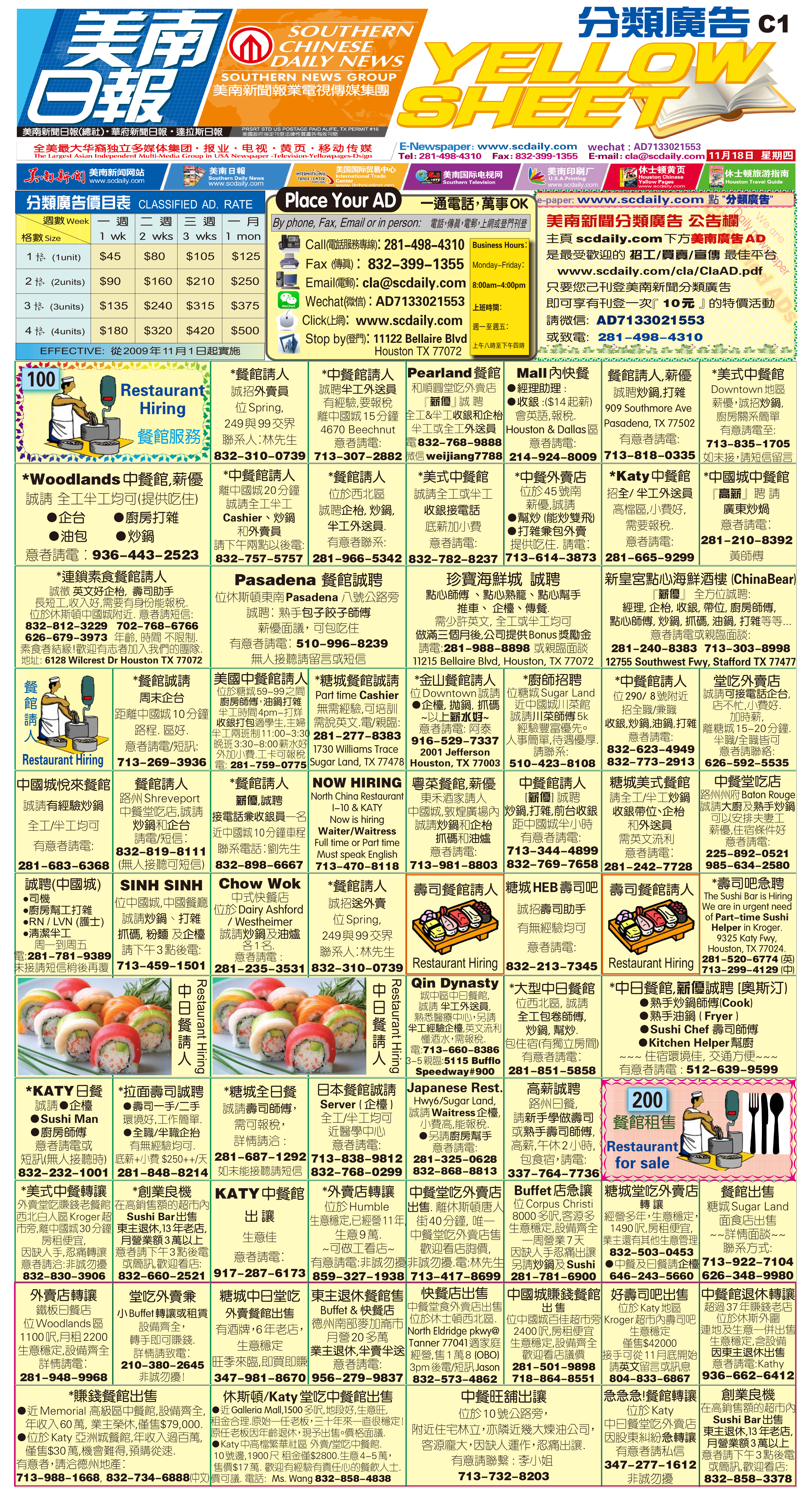211117 Epaper

 A Section B SectionC Section D Section211116 Epaper

 A Section B SectionC Section D Section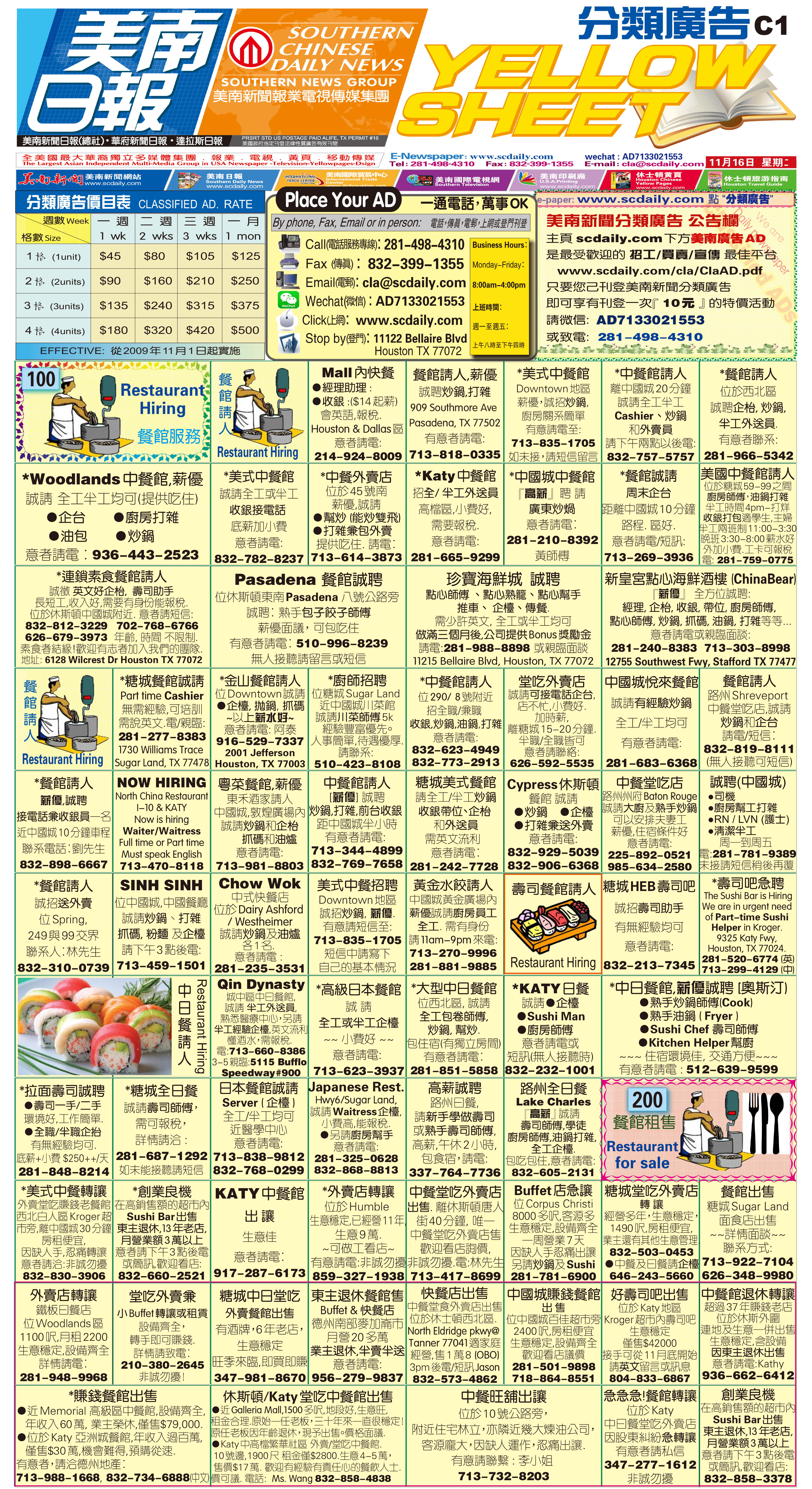211115 Epaper

 A Section B SectionC Section D Section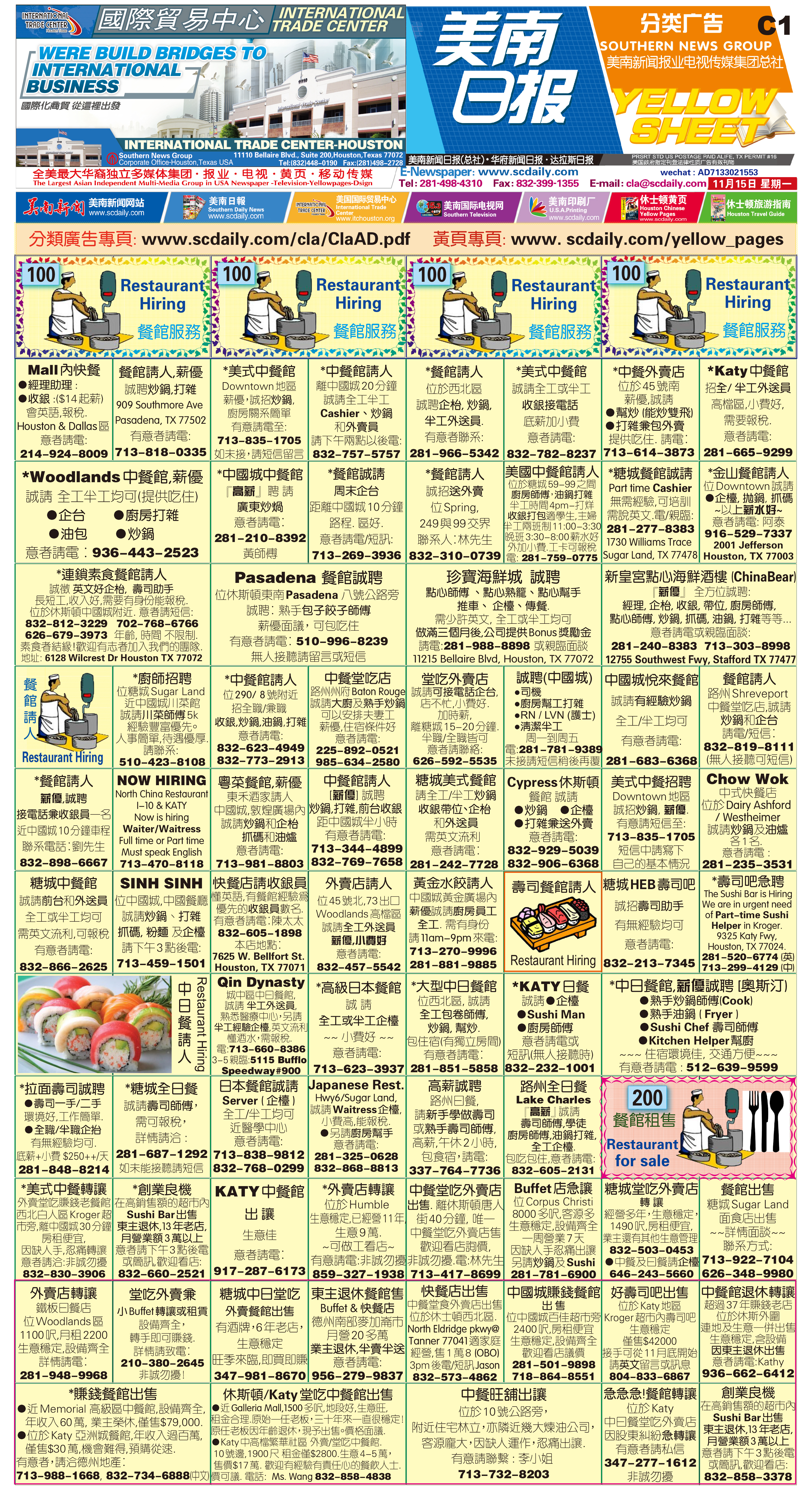211114 Epaper

 A Section B SectionC Section D Section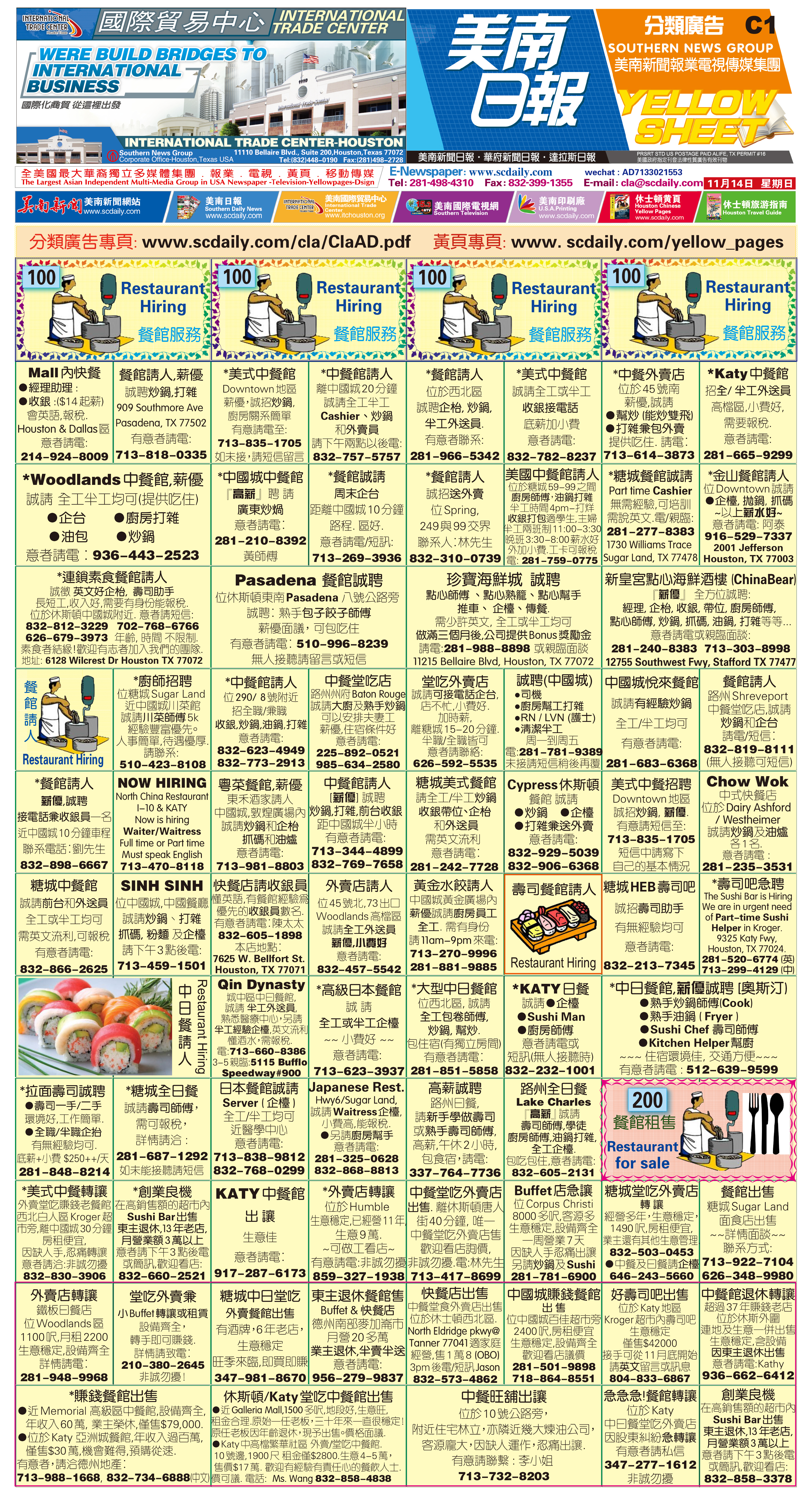211113 Epaper

 A Section B SectionC Section D Section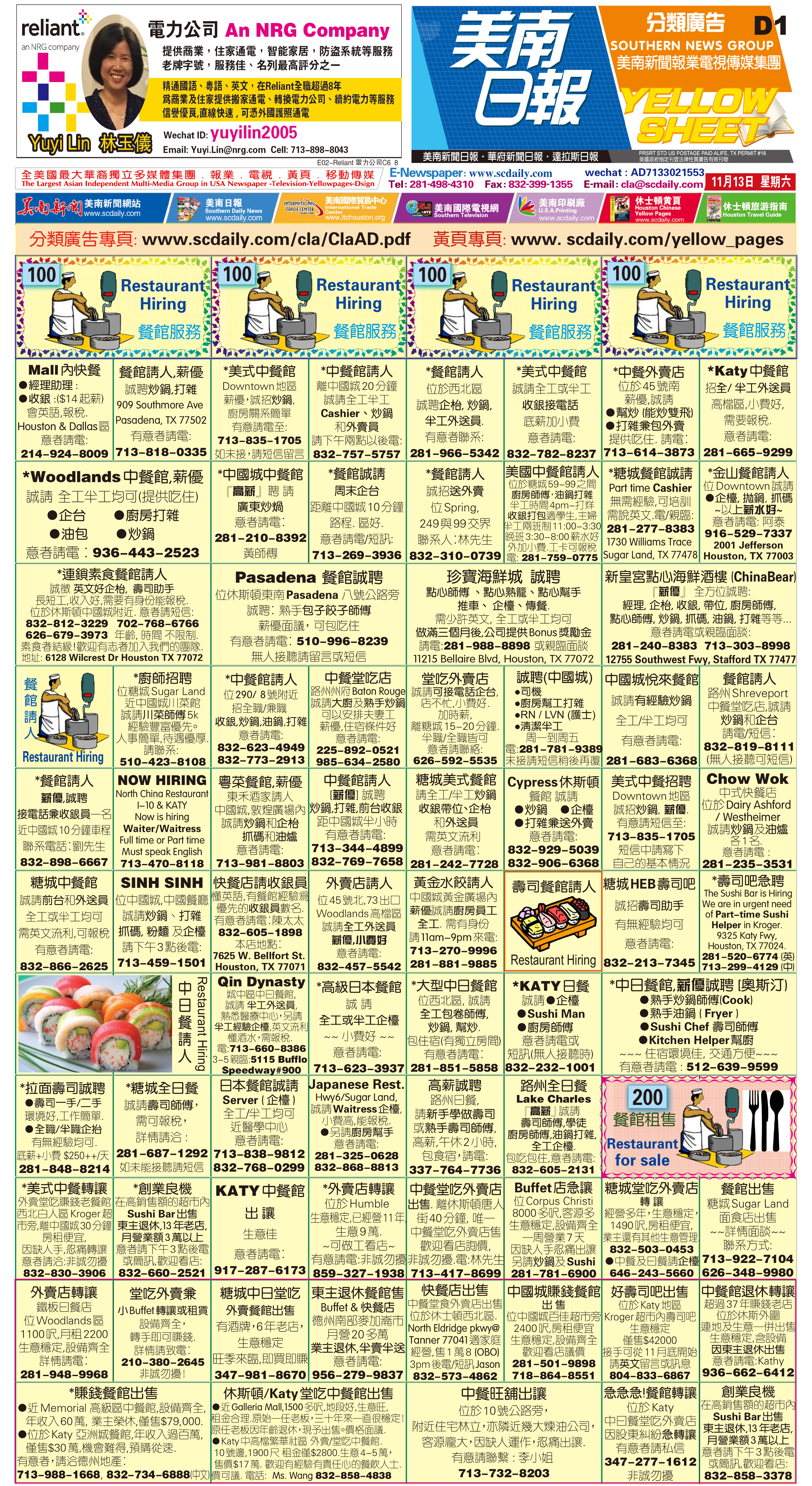211112 Epaper

 A Section B SectionC Section D Section211111 Epaper

 A Section B SectionC Section D Section211109 Epaper

 A Section B SectionC Section D Section211110 Epaper

 A Section B SectionC Section D Section211108 Epaper

 A Section B SectionC Section D Section